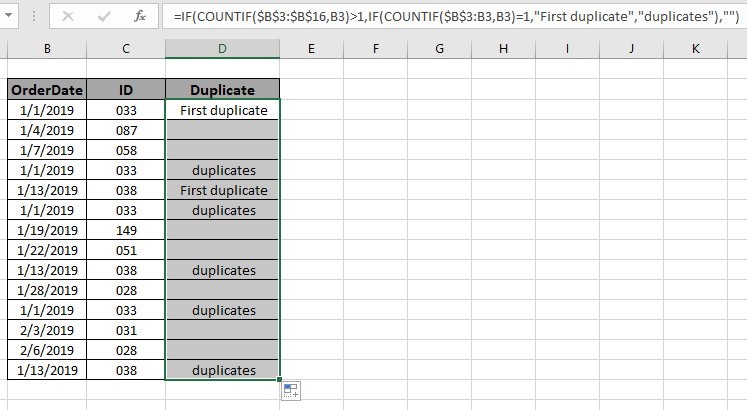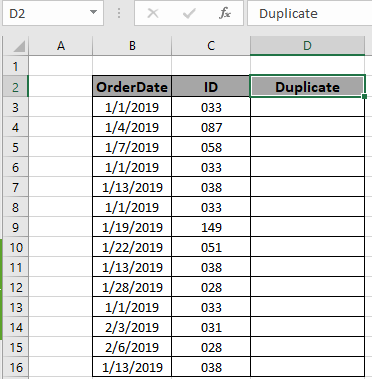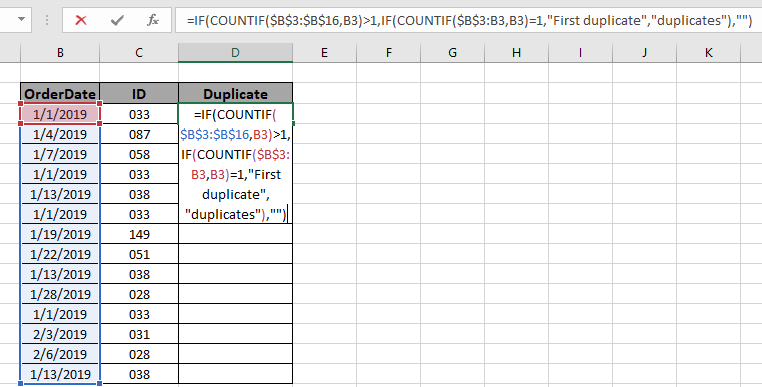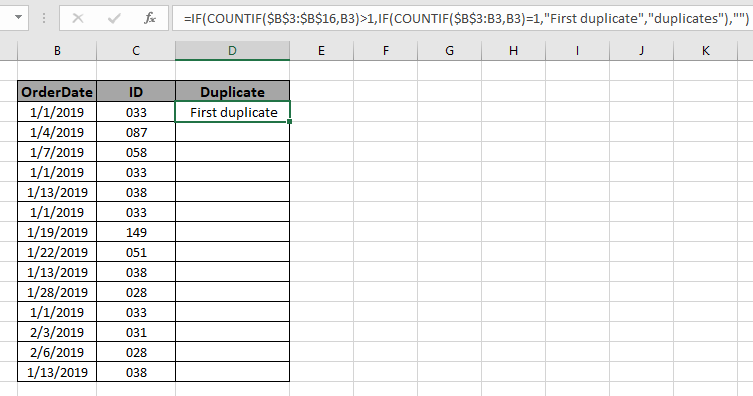# How to Mark First Duplicate in ExcelIn this article, we will learn how to flag the first duplicate in a range of values. Sometimes we need to identify the first duplicate from the other ones. Finding the first duplicate manually can be tedious or even impossible if  you have thousands of rows of data. Don't worry, we have a formula that can mark the first duplicate easily in seconds.

For this article we will be required to use the COUNTIF function and IF function together.

Generic formula:

= IF ( COUNTIF ( fixed_array , value ) > 1 , IF ( COUNTIF ( expending_array , value ) = 1 , "First duplicate" , "duplicates" ) , "" )

fixed array: fixed array of values.

Value: value for which we need to find the first duplicates that need to be found.

expending_array : Its a referencing type, that extends when copied down.

### Example: Find the first duplicates of IDs in the range.

Here we have the ID records with dates. We need to find the first and rest duplicates in the range separately.Here the arguments to the function are fed using the cell reference.

Use the formula:

= IF ( COUNTIF ( \$B\$3:\$B\$16 , B3 ) > 1 , IF ( COUNTIF ( \$B\$3:B3 , B3 ) = 1 , "First duplicate" , "duplicates" ) , "")

Explanation:

• COUNTIF function returns the count of the value in array.
• COUNTIF ( \$B\$3:\$B\$16 , B3 ) > 1, IF function checks the first condition to be the count be greater than 1 of value in B3 cell in array. If it stands TRUE, the IF function checks one more condition
• COUNTIF ( \$B\$3:B3 , B3 ) = 1, IF function checks the occurrence of value in B3 cells in absolute array reference.
• This condition can separate on the stated above two conditions and returns blank if unique value, "first duplicate" if first occurrence of "duplicates" for rest of the duplicate values.Here is the range that is given as array reference and value is given as cell reference. Press Enter to get the count.As you can see from the first value returns, the first duplicate means this value as other duplicates. Now copy the formula in other cells using the drag down option or using the shortcut key Ctrl + D as shown below.As you can see all the different duplicates are marked which proves the formula works fine.

Here are some observational notes shown below.

Notes:

1. The formula only works with both numbers and text both.
2. You can apply the formula over any column of the table where conditions can be applied.
3. Careful working with fixed reference and absolute array reference.
4. Operators like equals to ( = ), less than equal to ( <= ), greater than ( > ) or not equals to ( <> ) can be performed within function applied with numbers only.

Hope this article about how to flag the first duplicate from the range in Excel is explanatory. Find more articles on COUNTIF functions here. If you liked our blogs, share it with your friends on Facebook. And also you can follow us on Twitter and Facebook. We would love to hear from you, do let us know how we can improve, complement or innovate our work and make it better for you. Write us at info@exceltip.com

Related Articles

How to use the SUMPRODUCT function in Excel: Returns the SUM after multiplication of values in multiple arrays in excel.

COUNTIFS with Dynamic Criteria Range : Count cells dependent on other cell values in Excel.

COUNTIFS Two Criteria Match : Count cells matching two different criteria on list in excel.

COUNTIFS With OR For Multiple Criteria : Count cells having multiple criteria match using the OR function.

The COUNTIFS Function in Excel : Count cells dependent on other cell values.

How to Use Countif in VBA in Microsoft Excel : Count cells using Visual Basic for Applications code.

How to use wildcards in excel : Count cells matching phrases using the wildcards in excel

Popular Articles

50 Excel Shortcut to Increase Your Productivity : Get faster at your task. These 50 shortcuts will make you work even faster on Excel.

How to use the VLOOKUP Function in Excel : This is one of the most used and popular functions of excel that is used to lookup value from different ranges and sheets.

How to use the COUNTIF function in Excel : Count values with conditions using this amazing function. You don't need to filter your data to count specific values. Countif function is essential to prepare your dashboard.

How to Use SUMIF Function in Excel : This is another dashboard essential function. This helps you sum up values on specific conditions.

Terms and Conditions of use

The applications/code on this site are distributed as is and without warranties or liability. In no event shall the owner of the copyrights, or the authors of the applications/code be liable for any loss of profit, any problems or any damage resulting from the use or evaluation of the applications/code.